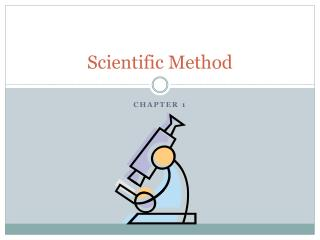DownloadDownload PresentationScientific Method

# Scientific Method

Download Presentation## Scientific Method

- - - - - - - - - - - - - - - - - - - - - - - - - - - E N D - - - - - - - - - - - - - - - - - - - - - - - - - - -
##### Presentation Transcript

1. Scientific Method Chapter 1

2. Scientific Method The scientific method is a series of steps used to solve problems. Steps: State a question Hypothesis Background Information Variables Experiment Gather data Conclusion

3. Why do we use the Scientific Method Using a series of steps allows for: Reliable results Unbiased results Consistency The scientific method can be used to solve any problem. Where is my science book? Who took the last cupcake? How can I get to class on time?

4. Scientific Method Problem: Where is my science book? Background info: Ms. Rodriguez’s gave me a book, I should have my science book. Hypothesis: Maybe it’s under the bed. Experiment: look under the bed. Analysis: It’s under the bed. Conclusion: I found my book, it was under the bed.

5. State a Question First step of the scientific method is to ask a question. Examples of a question could be: Will different amounts of sea salt affect the reproduction rate of sea monkeys.

6. Background Information • Background information is research about the topic of your experiment. All useful information about your topic should be included. Learning as much as possible about sea monkeys and their reproduction rate is useful before conducting your experiment. This allows for the formation of a good hypothesis.

7. Hypothesis A hypothesis is an educated guess on what you think might be the result of your experiment. IF100 ml of sea salt are added to a water solution containing 200 ml of sea salt and sea monkeys THENit will increase the rate at which sea monkeys reproduce by 10%

8. How to write a Hypothesis Hypothesis need to be written as cause and effect. IF__________________ THEN_______________ It should always state what you think might happened at the end of your experiment. It should also state measurements. If 200 ml of milk are added to lima bean plant A for 20 days then lima bean plant A will grow 5 cm higher than Lima bean plant B.

9. Variables When conducting an experiment to solve a problem or answer a question variables are used. Variables help to find the truest answer in an experiment. Variablesare factors that can change or stay the same. In the scientific method independent variable, dependent variable and control group are used.

10. Variables • Independent variable is a factor that is intentionally changed in the experiment. • Dependent variable changes depending on changes done to the independent variable.

11. Independent and Dependent Variables The variables of the sea monkey experiment are: Independent variable is the different amounts of salt added to each tank. Dependent variable is what changed as a result of the different amounts of salt, the reproduction rate.

12. Control and Constant • The control group is the group that is not changed by the independent variable. Control groups are used for comparison. In this case a tank that is not given a higher dose of sea salt. • A constant is a factor that stays the same in the experiment. With the sea monkey experiment it could be the amount of water in each individual tank and the amount of sea monkeys in each tank.

13. Experiment Next step: An experiment is using controlled conditions to test your hypothesis. In the sea monkey experiment the hypothesis stated that 100 ml of salt more than usual would increase the reproductive rate of sea monkeys. To test this hypothesis an experiment has to be conducted. Three tanks can be used in the experiment labeled 1, 2, and 3. Tank 1 is the control group. Tank 2 has 100 ml of sea salt. Tank 3 has 200 ml of sea salt.

14. Gathering Data • The next step is to gather data. Observe the experiment and write down the results. Then make graphs or tables to illustrate the results.

15. Final step: Conclusion The conclusionis the summary of the experiment, explaining if the hypothesis was correct. A conclusion must include: - Was the hypothesis accepted or rejected? • What where the results of the experiment? • What could have been done differently to improve the experiment?

16. Conclusion Sometimes the result of an experiment prove the hypothesis is incorrect. When this occurs, a new hypothesis is form and tested again.

17. Home learning Find a science fair topic Create a title for your project based on your topic Create the problem statement of your project.